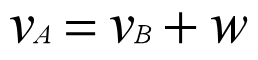Putting Newton's second law of motioninto two reference frames, we get:The second law of motion under Special Relativity

Under Special Relativity, the length, time, and mass all change along with their reference frames, so the equation above cannot be simplified any further.

The second law of motion under Newtonian system

In the Newtonian system, the length, time, and mass are the same in all reference frames, sobecomes:By Integration, we get:This is the normal velocity transformation formula.

So, in the Newtonian system, the first postulate and the velocity transformation formula are the same thing.